## Vibrational states and spectra of diatomic molecules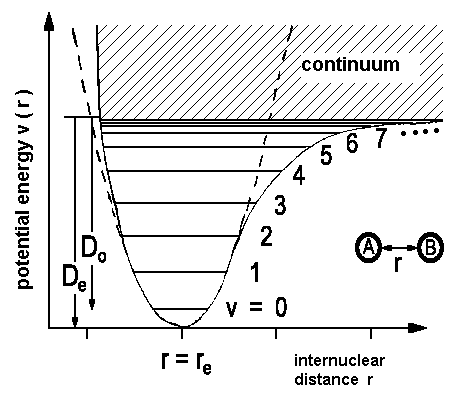In a previous chapter, we already used the energy levels and wave functions for an harmonic oscillator to understand diatomic molecules. Because changes of the electric dipole moment always occur when matter interacts with light, no vibrational spectra are found for homonuclear molecules. For heteronuclear molecules, the selection rules of the harmonic oscillator are applicable.
 Δv  =  ± 1 E  =  hω0(v + ½) v  =  0, 1, ...

### Origin of the selection rules for the harmonic oscillator

It is possible to expand the dipole moment µ with the internuclear distance as its origin. This distance, appearing as r in the potential diagram above, is denoted as variable z in the following mathematical treatment.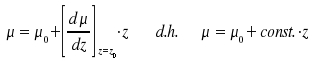The eigenfunctions of the harmonic oscillator are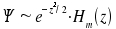. Thus, we obtain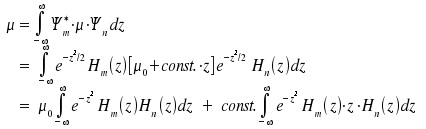Due to the orthogonality of the Hermite polynomials, the only situation where the first term contributes to µ is if m = n. This condition is incompatible with the fact that any transisition means that m ≠ n. To evaluate the second integral we use the following relation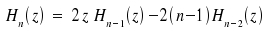Substitution n+1 for n and transformation yields the equation z · Hn(z) = ½ Hn+1(z) + n · Hn-1(z). By inserting into the second integral we obtain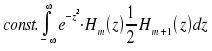+not equal zeroonly in cases where m = n + 1 not equal zeroonly in cases where m = n - 1

Therefore, a change of one for v is needed to have transitions between two states.

Improved mathematical descriptions of the vibrational states are based on the Morse potential V(r)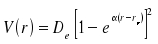where De is the minimal value of the potential and is found for x = xe and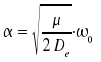With the potential curve, the energy levels obtained are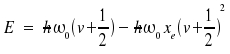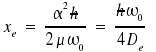Regrettably, the Morse potential cannot often be applied in many problems, Schroedinger's equation has to be solved anew. In practice, to calculate molecular constants from experimental data, an equation of the following type is used.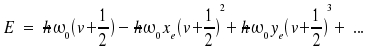Note that ωexe and ωeye are regarded as one single constant instead of a product. The counterpart of the distance between two levels is a line at some position within the spectrum. The energies respective transitions v + 1 ← v are: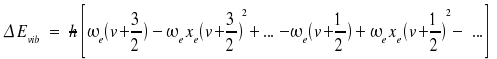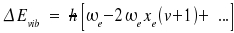The frequency for the so-called fundamental that reflects a transition v = 1 ← v = 0 therefore is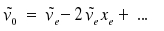The zero point energy Ev=0 appears shifted too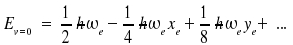Examples (wavenumbers in cm-1)
 molecule ~νe / cm-1 ~νexe / cm-1 ~ν0 / cm-1 re / pm remark H2 4401 121 4159 74,1 ← inactive within IR HCl 2991 52,8 2885 127,5 N2 2359 14,3 2330 109,8 ← inactive within IR I2 214 0,61 212,8 266,6 ← inactive within IR OH 3738 84,9 3568 96,97

As, compared with kT at room temperature, the vibrational energies generally are quite high. Transitions related to absorption only occur between v = 0 and v = 1. On the other hand, chemical reactions may form molecules in high vibrational levels and emissions from such levels need to be considered. For example, the origin of some weak luminescence in the upper stratosphere is an excited OH-radical emerging from the H + O3 → OH(v = 9) + O2.

Another two useful equations:
The molar bond dissociation enthalpy ΔHm and dissociation energy D0 are related by:NA: Avogadro's number

The difference between the dissociation energy D0 and the depth De of the potential equals the zero point energy.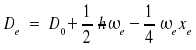Harmonics

Due to anharmonicity, spectra may display lines forbidden by the selection rule Δv = ± 1. The intensity of these harmonics is weaker. Due to lasers and their high energy flux, it has been possible to stimulate harmonics. The table below lists the spectral position of some harmonics of the OH radical. σ0 represents the relative intensity of the line; the fundamental line's intensity is set to 100.
 Example: Transition probabilities for the OH radical v' ← v'' ν / cm-1 λ / nm σ0 spectral range 1 ← 0 3568 2803 100 infrared 2 ← 0 6966 1436 16 3 ← 0 10195 980 0.5 4 ← 0 13253 755 0.03 visible 5 ← 0 16142 619.5 0.01

Vibrations of polyatomic molecules

Polyatomic molecules may vibrate in 3N-5 different ways if they are linear and in 3N-6 different ways if they are not. Therefore, the carbon dioxide molecule has four degrees of freedom for vibrations (the molecule can bend in two directions); whereas the water molecule has only three. Normal modes of vibration are mutually independent motions of groups of atoms; any one mode may be excited without leading to excitation of the others.

For more complex molecules it is advantageous to classify the normal modes of vibration according to symmetry in point groups (e.g. C2v for water). These aspects dictate whether vibrations are involved in transitions in the IR range of the spectrum, i.e. if the vibration is IR active.

The diagram below shows the vibrational states of the water molecule and the respective energies. Each state is IR-active and, of course, in none of them vibrational motions affect the centre of mass.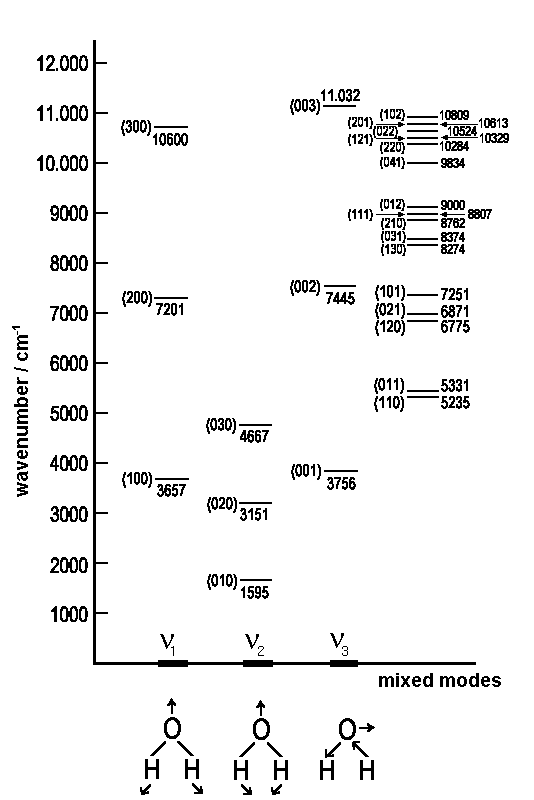Vibrational levels of gazeous water in cm-1. The numbers in brackets (v1,v2,v3) characterize the pure and mixed modes of vibrationHomeAuf diesem Webangebot gilt die Datenschutzerklärung der TU Braunschweig mit Ausnahme der Abschnitte VI, VII und VIII.